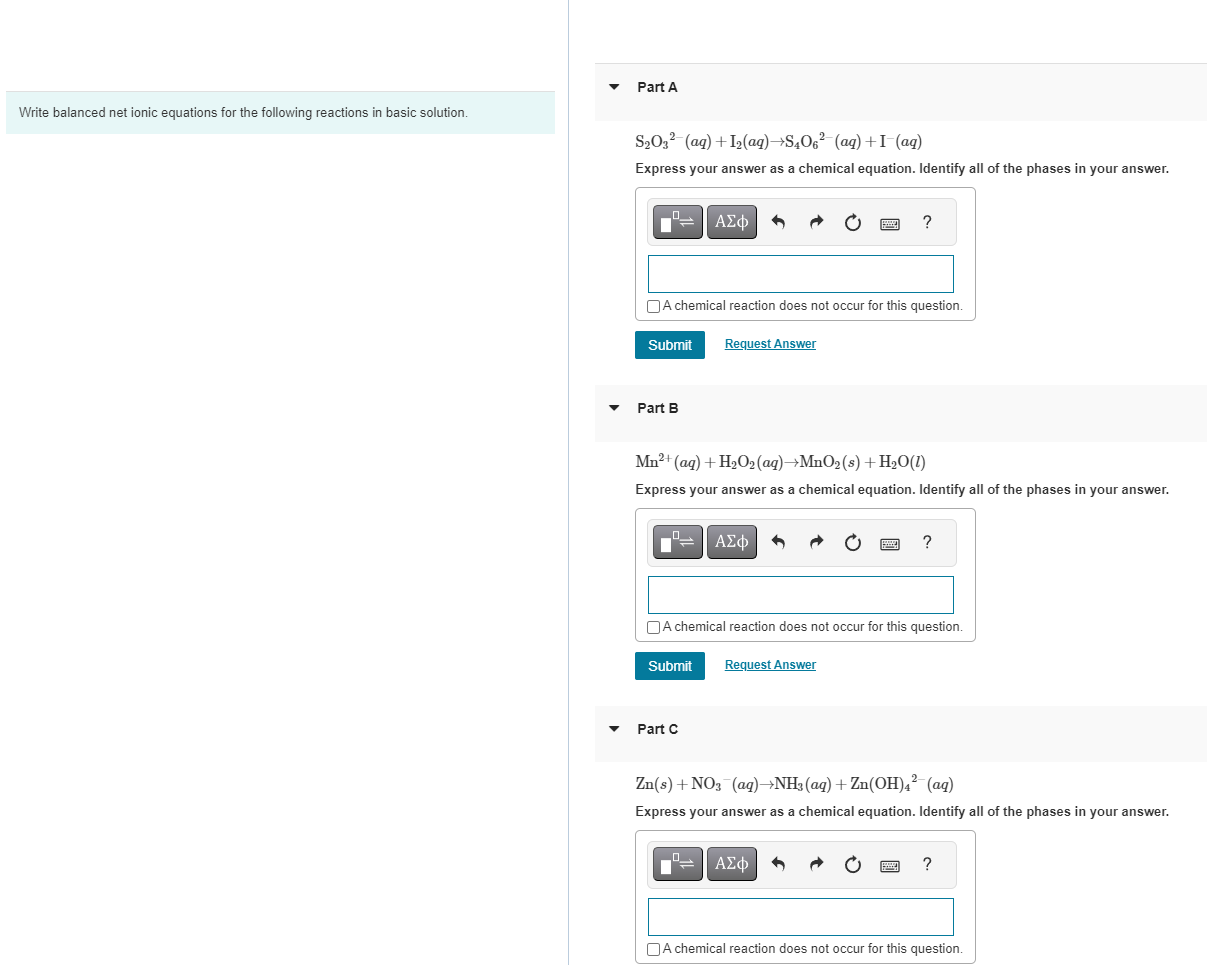Home / Expert Answers / Chemistry / write-balanced-net-ionic-equations-for-the-following-reactions-in-basic-solution-s2o32-aq-pa240

# (Solved): Write balanced net ionic equations for the following reactions in basic solution. S2O32(aq ...Write balanced net ionic equations for the following reactions in basic solution. Express your answer as a chemical equation. Identify all of the phases in your answer. A chemical reaction does not occur for this question. Part B Express your answer as a chemical equation. Identify all of the phases in your answer. A chemical reaction does not occur for this question. Part C

We have an Answer from Expert